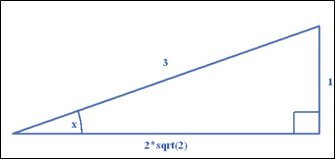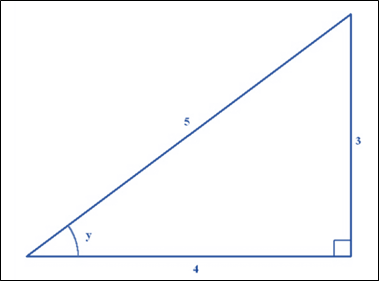# The value of following expression: sin ( x + y )### Single Variable Calculus: Concepts...

4th Edition
James Stewart
Publisher: Cengage Learning
ISBN: 9781337687805### Single Variable Calculus: Concepts...

4th Edition
James Stewart
Publisher: Cengage Learning
ISBN: 9781337687805

#### Solutions

Chapter C, Problem 27E
To determine

## To calculate: The value of following expression:   sin(x+y)

Expert Solution

The given expression sin(x+y) is evaluated as 4+6215 .

### Explanation of Solution

Given information:

sinx=13secy=540x,yπ2

Formula Used:

sin(x+y)=sinxcosy+cosxsiny

Calculation:

Know that:

sinx=oppositehypotenussinx=13

First need to sketch the triangle for sinx :Fig. 1 Triangle for sinx

So, hypotenuse is equal to 3 and the length of the adjacent side is equal to 1 . So, use the Pythagoras theorem:

Know that:

Need to sketch the triangle for secx :Fig. 2 Triangle for secx

So, hypotenuse is equal to 5 and the length of the adjacent side is equal to 4 . So, use the Pythagoras theorem:

r2=opposite2+a252=opposite2+42opposite2=9opposite=3

And the remaining side opposite to y is 3 .

From first triangle:

sinx=13cosx=223

From second triangle:

siny=35cosy=45

Now, evaluate the given expression as:

sin(x+y)=sinxcosy+cosxsinysin(x+y)=(13)(45)+(223)(35)sin(x+y)=415+6215sin(x+y)=4+6215

### Have a homework question?

Subscribe to bartleby learn! Ask subject matter experts 30 homework questions each month. Plus, you’ll have access to millions of step-by-step textbook answers!